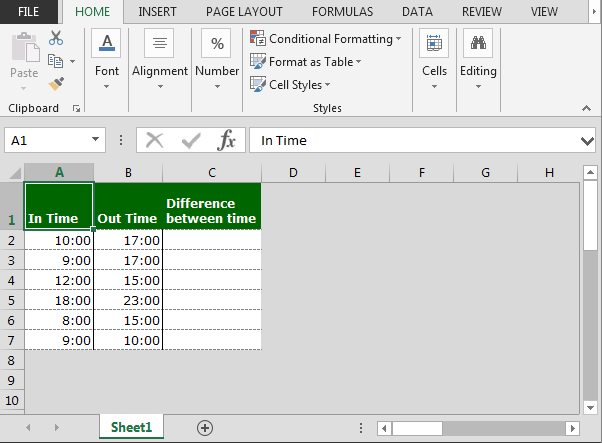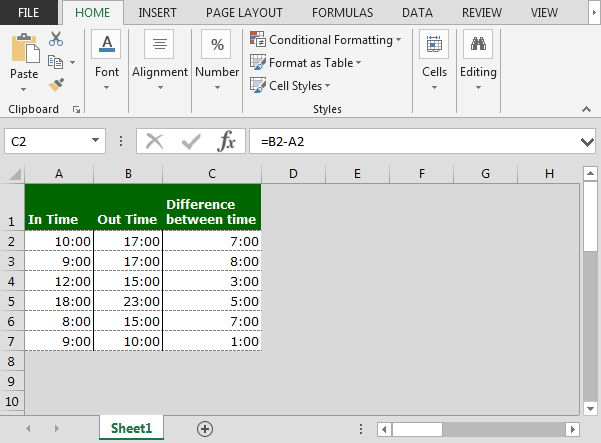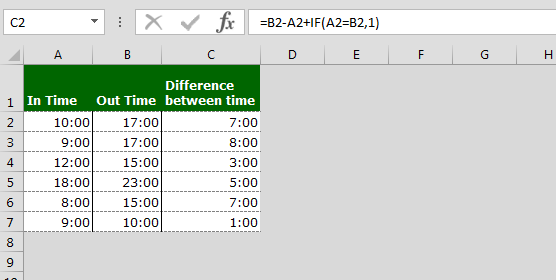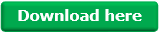Calculating the Difference between Hours in Microsoft Excel

Before understanding how we will calculate the difference between times, we need to understand that Excel stores the time information as number in decimal values. To convert the number into time, we have to format the number in time format.

So to calculate the difference between hours, we just subtract the time in the same way as we calculate the numbers. Whenever we face the error problem while calculating the time, these problems take place due to wrong formatting.

Let’s take an example and understand how we can calculate the difference between times:-

We have 2 times- In time and Out time. In column C, we want to return the difference between In time and Out time. Overall, we want to calculate the working hours.Follow the below given steps:-

• Enter the formula in cell C2.
• Subtract the both cells =B2-A2.
• Press Enter on your keyboard.
• The function will return the time difference between two times.
• Copy the same formula and paste in the range C3:C7.This is the way we can return the difference between the times.

Also, we can use below mentioned formula:-

• The number 1 in the IF formula equals 24 when working with time.
• For example, in row 6, the calculation is 6.00 – 20.31+ 24.00 = 9.29.Download – Calculate Time Difference in Excel – xlsx

## Users are saying about us...

1.Naga Murali Mohan Chopperla

The info is helpful. Can you please help me with the calculations for 24hrs operation organisation

Example:

In time – 23:00 and Out time – 4:00 the difference is 5:00 and need in decimals as 5.00

Regards,

Naga Murali

•Hi Naga Murali,

You can use the below formula for your calculation. After applying the formula, change the cell’s formatting to number formatting.
=(B2-A2+IF(A2>=B2,1))*24

Thanks,
Team Excel Tip

Terms and Conditions of use

The applications/code on this site are distributed as is and without warranties or liability. In no event shall the owner of the copyrights, or the authors of the applications/code be liable for any loss of profit, any problems or any damage resulting from the use or evaluation of the applications/code.